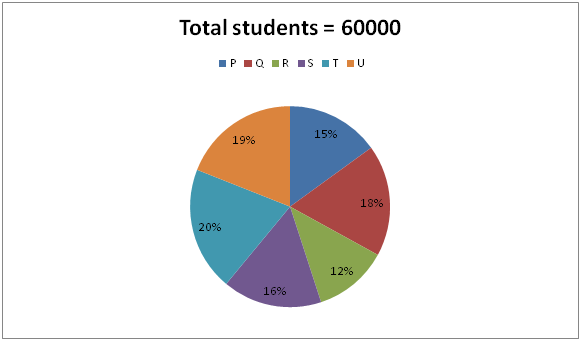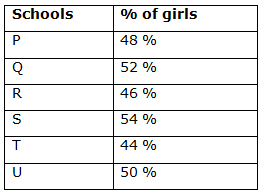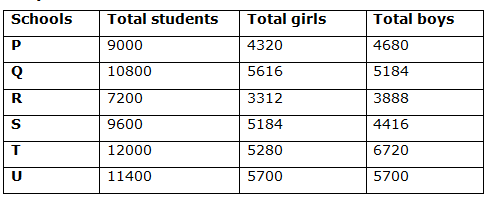# IBPS Clerk Prelims 2018 – Quantitative Aptitude Questions Day-17

Dear Readers, Bank Exam Race for the Year 2018 is already started, To enrich your preparation here we have providing new series of Practice Questions on Quantitative Aptitude – Section. Candidates those who are preparing for IBPS Clerk Prelims 2018 Exams can practice these questions daily and make your preparation effective.

[WpProQuiz 4161]

1) A man takes to row 120 km along the stream is one-third of the time taken to row against the stream. Find the speed of the boat in still water if the speed of stream is 8 km/hr?

a) 18 km/hr

b) 20 km/hr

c) 16 km/hr

d) 14 km/hr

e) None of these

2) An article was sold at a discount of 25 % and there was a profit of 20 %. If the profit earned was Rs. 100 less than the discount offered, then find the selling price of the article?

a) Rs. 650

b) Rs. 700

c) Rs. 750

d) 600

e) None of these

3) A basket contains 7 White, 5 Black, 2 Pink and 4 Green balls. If three balls picked up random, then find the probability that all three are Green balls?

a) 1/204

b) 15/157

c) 37/218

d) 23/512

e) None of these

4) 5 years ago, the ages of Agila and Hema are in the ratio of 3: 2. Three years ago, Agila’s age is equal to Hema’s age after 2 years. Then find the present age Agila?

a) 18 years

b) 24 years

c) 26 years

d) 20 years

e) None of these

5) A can do a piece of work in 24 days; B can do it in 30 days. They started the work together but A left 6 days before the completion of the work. The work has finished in?

a) 12 days

b) 15 days

c) 50/3 days

d) 25/3 days

e) None of these

Directions (Q. 6 – 10) Study the following information carefully and answer the given questions.

The following pie chart shows the total number of students in 6 different schools and the table shows the percentage of girls out of the total number of students in the respective schools.6) Find the difference between the total number of boys in School P to that of total number of girls in School R?

a) 1456

b) 1892

c) 1368

d) 1575

e) None of these

7) Find the average number of girls in School P, Q and S together?

a) 5040

b) 5180

c) 4890

d) 4560

e) None of these

8) Find the ratio between the total number of students in School R to that of total number of boys in School T?

a) 8: 7

b) 32: 29

c) 23: 21

d) 15: 14

e) None of these

9) The total number of girls in School U is approximately what percentage of total number of boys in School Q?

a) 95 %

b) 110 %

c) 135 %

d) 70 %

e) 120 %

10) Find the central angle for School T?

a) 660

b) 800

c) 720

d) 840

e) None of these

Let us take the speed of the boat in still water be x km/hr,

120/(x+8) = (1/3)*(120/(x-8))

3x – 24 = x + 8

2x = 32

X = 16

Speed of the boat in still water = 16 km/hr

Let the cost price be x,

Selling price = (120/100)*x = 6x/5

Marked price*(75/100) = 6x/5

MP = 8x/5

The profit earned was Rs.100 less than the discount,

According to the question,

= > (8x/5 – 6x/5) – (6x/5 – x) = 100

= > 2x/5 – x/5 = 100

= > x/5 = 100

Cost price = Rs. 500

Selling price = 500*(120/100) = Rs. 600

Total Balls = 18

Required Probability = 4C3 / 18C3 = 1/204

5 years ago, Agila: Hema = 3: 2 (3x, 2x)

Present ages of Agila and Hema = 3x + 5, 2x + 5

Three years ago, Agila’s age is equal to Hema’s age after 2 years,

= > 3x + 2 = 2x + 7

= > x = 5

Present age of Agila = 3x + 5 = 20 years

(x – 6)/24 + x/30 = 1

(30x – 180 + 24x)/720 = 1

54x – 180 = 720

54x = 900

X = 900/54 = 50/3 days

Direction (6-10) :The total number of boys in School P

= > 60000*(15/100)*(52/100) = 4680

The total number of girls in School R

= > 60000*(12/100)*(46/100) = 3312

Required difference = 4680 – 3312 = 1368

The total number of girls in School P, Q and S together

= > 60000*(15/100)*(48/100) + 60000*(18/100)*(52/100) + 60000*(16/100)*(54/100)

= > 4320 + 5616 + 5184 = 15120

Required average = 15120/3 = 5040

The total number of students in School R

= > 60000*(12/100) = 7200

The total number of boys in School T

= > 60000*(20/100)*(56/100) = 6720

Required ratio = 7200: 6720 = 15: 14

The total number of girls in School U

= > 60000*(19/100)*(50/100) = 5700

The total number of boys in School Q

= > 60000*(18/100)*(48/100) = 5184

Required % = (5700/5184)*100 = 110 %

The central angle for School T

= > (20/100)*360 = 720

Daily Practice Test Schedule | Good Luck

 Topic Daily Publishing Time Daily News Papers & Editorials 8.00 AM Current Affairs Quiz 9.00 AM Current Affairs Quiz (Hindi) 9.30 AM IBPS Clerk Prelims – Reasoning 10.00 AM IBPS Clerk Prelims – Reasoning (Hindi) 10.30 AM IBPS Clerk Prelims – Quantitative Aptitude 11.00 AM IBPS Clerk Prelims – Quantitative Aptitude (Hindi) 11.30 AM Vocabulary (Based on The Hindu) 12.00 PM IBPS PO Prelims – English Language 1.00 PM SSC Practice Questions (Reasoning/Quantitative aptitude) 2.00 PM IBPS PO/Clerk – GK Questions 3.00 PM SSC Practice Questions (English/General Knowledge) 4.00 PM Daily Current Affairs Updates 5.00 PM IBPS PO Mains – Reasoning 6.00 PM IBPS PO Mains – Quantitative Aptitude 7.00 PM IBPS PO Mains – English Language 8.00 PM